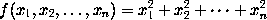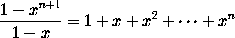### Ellipsis (i.e., three dots')

Ellipsis (three dots) is produced in mathematics mode using the control sequences \ldots (for dots aligned with tbe baseline of text), and \cdots (for dots aligned with the centreline of mathematical formulae). Thus the formulais obtained by typing
$f(x_1, x_2,\ldots, x_n) = x_1^2 + x_2^2 + \cdots + x_n^2$

Similarly the formulais produced using \cdots, by typing
$\frac{1 - x^{n+1}}{1 - x} = 1 + x + x^2 + \cdots + x^n$
`